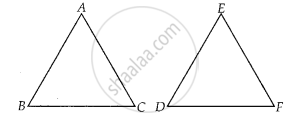# If in triangles ABC and DEF, ABDE=BCFD, then they will be similar, when ______ - Mathematics

MCQ
Fill in the Blanks

If in triangles ABC and DEF, (AB)/(DE) = (BC)/(FD), then they will be similar, when ______.

• ∠B = ∠E

• ∠A = ∠D

• ∠B = ∠D

• ∠A = ∠F

#### Solution

If in triangles ABC and DEF, (AB)/(DE) = (BC)/(FD), then they will be similar, when ∠B = ∠D.

Explanation:

Given, in ∆ABC and ∆EDF,

(AB)/(DE) = (BC)/(FD)So, ∆ABC ~ ∆EDF if ∠B = ∠D   ......[By SAS similarity criterion]

Concept: Criteria for Similarity of Triangles
Is there an error in this question or solution?

#### APPEARS IN

NCERT Mathematics Exemplar Class 10
Chapter 6 Triangles
Exercise 6.1 | Q 10 | Page 62
Share